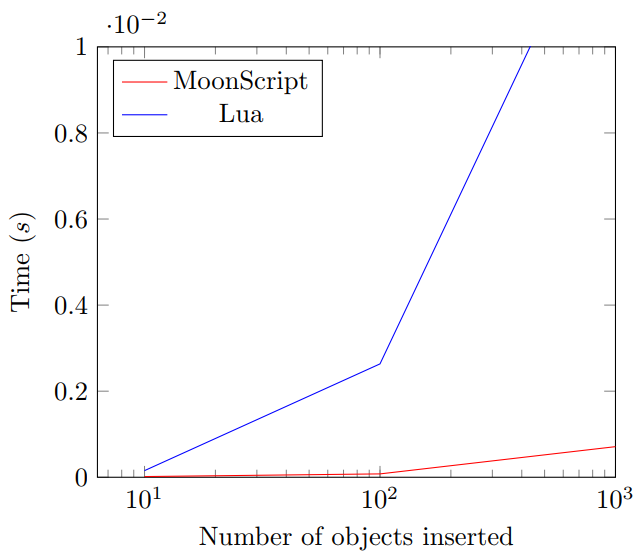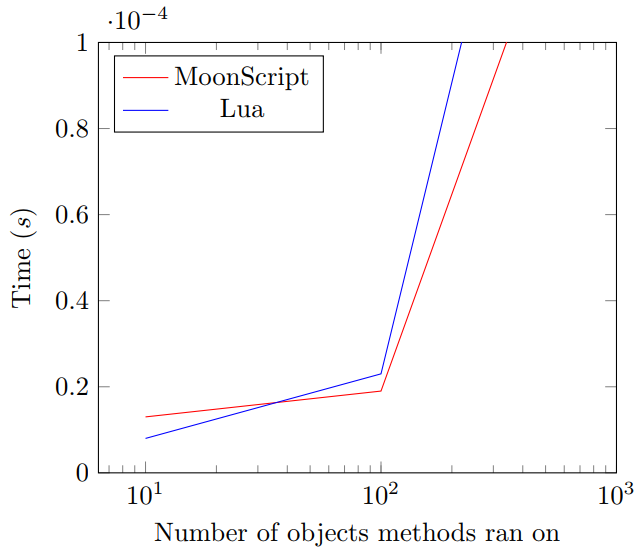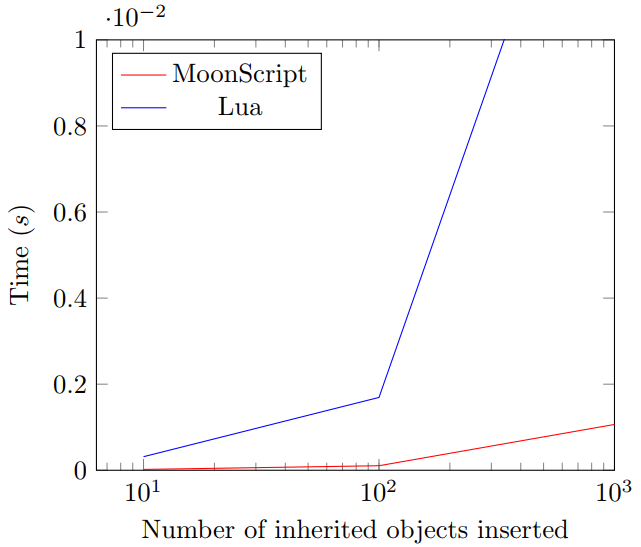# MoonScript vs Lua: A speed test

2017-11-19 20:39, 2 years and 2 weeks ago
5327900a40d3a8119a6fa523bed240f2

I’ve discovered MoonScript in the last month and so far I’m loving it (apart from a few whitespacing quirks). One of the things I like most about it is the built-in Object Orientation, something that is lacking from Lua (but can be added with libraries/metatables).

I’ve been interested in how fast the generated Lua code is from MoonScript for a while now, so today I’m going to do a little speed-test of sorts. This won’t be an incredibly indepth comparison - so don’t jump down my throat if I’ve fucked up somewhere. Feel free to correct me though.

I’ll be comparing speed differences from MoonScript OO to a minimal and fast Lua OO library, 30log.

The main 3 pillars of Object Orientation are: Objects, Methods and Inheritance. I’ll be seeing how long it takes each implementation to create these in increasing sizes.

## Creating objects

Simply defining a class called X, and creating and inserting ever-increasing amounts of X, from 10 to 1,000,000 instances.

  --lua
local class = require '30log'
t = {}
X = class("X")
Start = os.clock()
for i=1, 100 do table.insert(t, X:new()) end
End = os.clock()
print(End-Start)

  --moonscript
t = {}
class X
new: () =>
Start = os.clock()
for i=1, 100 do table.insert(t, X!)
End = os.clock()
print(End-Start)| No. of objects created | Time taken (s)         |
|------------------------|------------|-----------|
|                        | MoonScript | Lua       |
| 10                     | 1.6e-05    | 0.000154  |
| 100                    | 7.8e-05    | 0.002634  |
| 1,000                  | 0.000711   | 0.014191  |
| 10,000                 | 0.006792   | 0.144497  |
| 100,000                | 0.072799   | 1.493317  |
| 1,000,000              | 0.708374   | 16.057709 |


As you can see, the time taken for 30log to create objects increases massively to 16 seconds! - Although realistically most people will have less than 1,000 objects, the speed difference is still pretty large.

## Methods

Giving X an method called add which simply increments its self.x.

  t = {}
local class = require '30log'
X = class("X")
function X:init(x) self.x = x end
function X:add() self.x = self.x + 1 end
for i=1, 10 do table.insert(t, X(0)) end
Start = os.clock()
for i=1, #t do t[i]:add() end
End = os.clock()
print(End-Start)

  t = {}
class X
new: (@x) =>
@x += 1
for i=1, 100000 do table.insert(t, X(0))
Start = os.clock()
for x in *t do x\add()
End = os.clock()
print(End-Start)| No. methods ran | Time taken (s)        |
|-----------------|------------|----------|
|                 | MoonScript | Lua      |
|-----------------|------------|----------|
| 10              | 1.3e-05    | 7.98e-06 |
| 100             | 1.9e-05    | 2.3e-05  |
| 1,000           | 0.000171   | 0.000248 |
| 10,000          | 0.001755   | 0.00275  |
| 100,000         | 0.017076   | 0.029891 |
| 1,000,000       | 0.172534   | 0.312667 |


MoonScript is initially slower when object count is <100, but soon to lag starts to mount with 30log.

## Inheritance

Creating another class called Y that is inherited from X, we should expect similar results to the first test.

local class = require '30log'
t = {}
X = class("X")
function X:init(x) self.x = x end
function X:add() self.x = self.x + 1 end
Y = X:extend("Y")
function Y:init(x) self.x = x end
Start = os.clock()
for i=1, 10 do table.insert(t, Y(0)) end
End = os.clock()
print(End-Start)

  t = {}
class X
new: (@x) =>
@x += 1
class Y extends X
new: () =>
Start = os.clock()
for i=1, 100000 do table.insert(t, Y(0))
End = os.clock()
print(End-Start)| No. of objects inherited | Time taken | (s)       |
|--------------------------|------------|-----------|
|                          | MoonScript | Lua       |
| 10                       | 1.9e-05    | 0.000315  |
| 100                      | 0.000105   | 0.001692  |
| 1,000                    | 0.001064   | 0.017323  |
| 10,000                   | 0.01217    | 0.165252  |
| 100,000                  | 0.109585   | 1.721108  |
| 1,000,000                | 1.042791   | 17.100362 |


MoonScript is the clear victor here, 30log takes up to 17 seconds to complete the same task as MoonScript.

So yeah. I guess we can say MoonScript OO is faster then 30log OO (and also nicer looking). 30log is still a good OO library though, it provides a simple and fairly fast way to do OO programming without the need for pre-compiling.